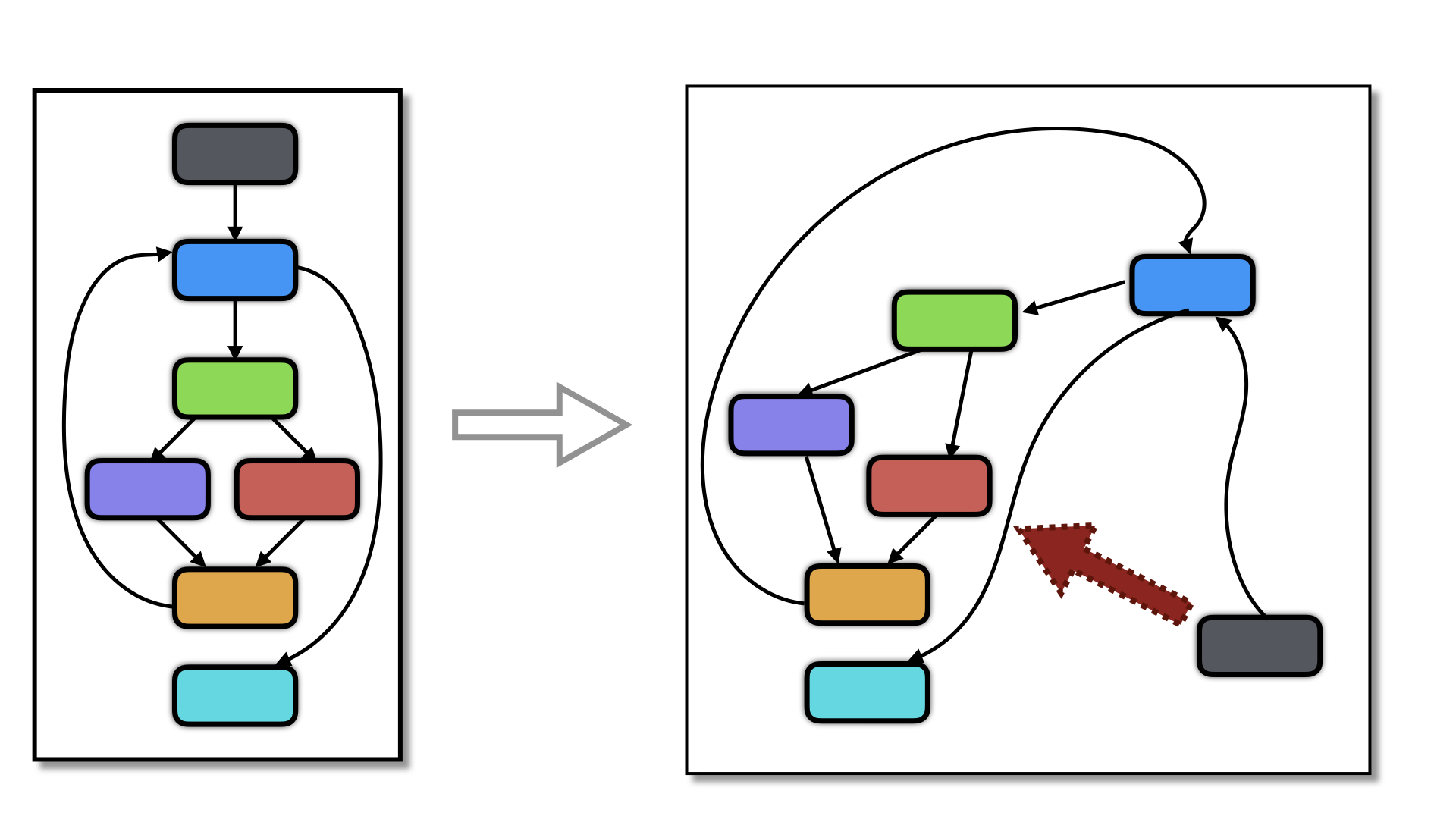# Self Modifyfree web templates

The goal of these transformations is to make functions self-modifying. Here's how Tigress is invoked:

``````> tigress  --Environment=x86_64:Darwin:Clang:5.1 \
--Transform=Virtualize\
--VirtualizeDispatch=direct \
--Transform=SelfModify \
--SelfModifyFraction=%100 \
--SelfModifySubExpressions=false \
--SelfModifyOperators=\* \
--SelfModifyKinds=\* \
--SelfModifyBogusInstructions=0 \
inc.c --out=obf.c
``````
free web templates

Note that we need to compile the generated code in such a way that the text segment pages are writable (See here for alternatives and here for issues with newer versions of Darwin):

``````> gcc -segprot __TEXT rwx rwx obf.c -o obf.exe   # Darwin (early versions)

> tigress_post --Action=writable obf.exe         # Darwin (newer versions)

> gcc --static -Wl,--omagic obf.c -o obf.exe     # Linux
``````

### Binary Arithmetic Expressions and Comparisons

free web templates

The transformation inserts a binary code template at the top of the function. There's one template for each type (int/long) and one for arithmetic, one for comparisons, and one for branches. They look roughly like this:

``````  addrPtr10 = (unsigned long *)((unsigned long )(&& Lab_1) + 5);
*addrPtr10 = (unsigned long )(& A);
addrPtr10 = (unsigned long *)((unsigned long )(&& Lab_1) + 18);
*addrPtr10 = (unsigned long )(& B);
addrPtr10 = (unsigned long *)((unsigned long )(&& Lab_1) + 41);
*addrPtr10 = (unsigned long )(& C);
addrPtr10 = (unsigned long *)((unsigned long )(&& Lab_1) + 61);
*addrPtr10 = (unsigned long )(&& Lab_2);
Lab_1:
__asm__  volatile   (
".byte 0x50;\n"             // push %eax/rax
".byte 0x51;\n"             // push %ecx/rcx
".byte 0x56;\n"             // push %rsi/esi
".byte 0x48, 0xb8,0xc,0x7b,0x21,0x3d,0x54,0x2d,0xc0,0x1;\n"   //movabs &A,%rax
".byte 0x8b, 0x00;\n"       // movl (%rax),%eax
".byte 0x48, 0xb9,0x4f,0x40,0x2f,0xa3,0xd0,0xdc,0x24,0x42;\n" //movabs &B,%rcx
".byte 0x8b, 0x09;\n"       // (%rcx),%ecx
".byte 0x31, 0xf6;\n"       // xorl  %rsi,%rsi
".byte 0x39, 0xc8;\n"       // cmpl  %rcx, %rax
".byte 0x40, 0x0f, 0x9c, 0xc6;\n" // setl  %sil
".byte 0x48, 0xb9,0x7f,0x91,0x1c,0xaf,0xab,0x5f,0x2d,0x6a;\n" // movabs &C,%rcx
".byte 0x67, 0x89, 0x31;\n" // movl %esi,(%rcx)
".byte 0x5e;\n"             // popq %rsi/esi
".byte 0x59;\n"             // popq %ecx/rcx
".byte 0x58;\n"             // popq %eax/rax
".byte 0xff, 0x25, 00, 00, 00, 00, 0xba,0xee,0x19,0x51,0x13,0x4b,0x12,0xdb;\n" // jmp (%rip)
:);
Lab_2:
``````
free web templates

Then, for each binary operation in the remainder of the function, the following code is inserted:

``````    A = i;
B = n;
addrPtr10 = (unsigned long *)((unsigned long )(&& Lab_3) + 6);
*addrPtr10 = (unsigned long )(&& Lab_1);
addrPtr10 = (unsigned long *)((unsigned long )(&& Lab_1) + 61);
*addrPtr10 = (unsigned long )(&& Lab_4);
opPtr11 = (int *)((unsigned long )(&& Lab_1) + 35);
*opPtr11 = 0xc69c0f40L;    // setl   %sil
Lab_3:
__asm__  volatile   (
".byte 0xff, 0x25, 00, 00, 00, 00, 0x3b,0x21,0x2e,0xd7,0xc5,0x44,0xf0,0xdd;\n" //jmp (%rip)
:);
Lab_4:
``````
free web templates

At runtime, this makes the following changes to the executable code:

• the opcode is set to the appropriate one (setl in this case),
• the jump instruction is modified with the literal address of the template (Lab_1),
• the return jump instruction in the template (the last instruction) is set to the return location (Lab_4).

### Virtualization/Flattening + Self-Modification

free web templates

This transformation is particularly useful after transformations that introduce indirect branches, such as virtualization and flattening with a direct or indirect dispatch. Below is an example:

``````void add(void) {
...

//----------------- Instruction Handler ----------------------

// BEGIN indirect branch to the next instruction starts here
branchAddr10 = (unsigned long *)((unsigned long )(&& Lab_3) + 6);
Lab_3: /* CIL Label */
__asm__  volatile   (".byte 0xff, 0x25, 00, 00, 00, 00, 00, 00, 00, 00, 00, 00, 00, 00":);
// END

//----------------- Instruction Handler ----------------------
return;
...
}
``````
free web templates

Here, each indirect branch turns into the following code, where the byte sequence corresponds to the X86 8-byte direct jump:

``````  addr = (unsigned long *)((unsigned long )(&& Lab) + 6);
Lab: asm volatile(".byte 0xff, 0x25, 00, 00, 00, 00, 00, 00, 00, 00, 00, 00, 00, 00":);
``````
free web templates

This is interesting, since some de-virtualization transformations look for indirect branches, and these are now gone from the code.

OptionArgumentsDescription
--Transform SelfModify Transform the function by adding code that modifies itself.
--SelfModifyKinds indirectBranch, arithmetic, comparisons Types of instructions to self-modify. Default=indirectBranch.
• indirectBranch = Transform indirect branches
• arithmetic = Transform binary arithmetic expressions
• comparisons = Transform binary comparisons
--SelfModifyFraction FRACSPEC How many statements should be self-modified. Default=%100.
--SelfModifySubExpressions BOOLSPEC Recurse into sub-expressions with transforming an expression. Can cause bugs. Default=false.
--SelfModifyBogusInstructions PlusA, MinusA, Mult, Div, Mod, Shiftlt, Shiftrt, Lt, Gt, Le, Ge, Eq, Ne, * Which binary operators to modify. Default=*.
• PlusA = +
• MinusA = -
• Mult = *
• Div = /
• Mod = %
• Shiftlt = <<
• Shiftrt = >>
• Lt = <
• Gt = >
• Le = =<
• Ge = >=
• Eq = ==
• Ne = !=
• * = all operators
--SelfModifyBogusInstructions INTSPEC How many bogus instructions to insert inside the self-modify template to avoid pattern-matching attacks. Right now, the inserted instructions are simple 1-byte x86 instructions that are equivalent to NOPs. Default=0.

### Issues

• This transformation currently only works on x86/64 targets.
• If you see an error message "Simplify: makeThreeAddress for AddrOf", set --SelfModifySubExpressions=false There's a bug in CIL that I don't know how to fix.
• At this time, there is not much diversity in generated code; it's very tamplate-y. This makes it easy to locate the code using simple pattern-matching. To break up the templates somewhat, set --SelfModifyBogusInstructions=0?5. This inserts between 0 and 5 NOP-equivalent bytes between the template instructions.